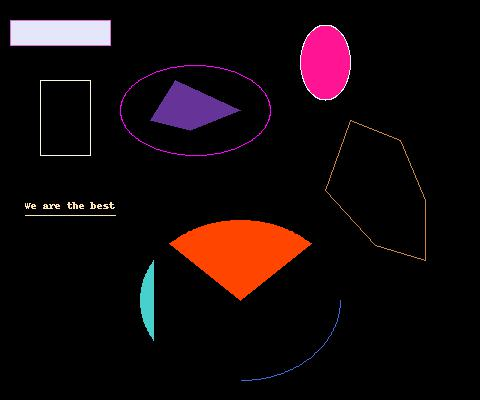## Pillow

• The primary package for support graphics and image manipulation is Pillow. Pillow is not part of the standard Python install; you have to add it yourself. To install Pillow on your laptop go here. The parts of Pillow of interest to us are:
• Image : Pillow representation of an image.
• To gain access to Pillow functionality the module `PIL` needs to be imported. The typical way to gain access to `Image` and `ImageDraw` is statement

from PIL import Image, ImageDraw

### Image

• The Image function that we care about for now is its `new()` function — through it we can construct a new image.
• Function invocation `Image.new( m, size, color='Black' )` returns a mode `m` new image, whose dimensions are `size`; where the background of the image is filled-in with `color`. Parameter `color` is optional with a default value of black. The units of measure for the size are in pixels. For us, `m` will always be the string `'RGB'`; this mode uses differents levels of red, green, and blue to produce other colors.• Parameter `size` will always be an ordered pair of the form `( w, h )`, where `w` is the width of the image and `h` is the height of the image.
• The following code segment defines a new image object called `im`. The image is 550 pixels wide and 450 pixels high and its background color is midnight blue.

im_width = 480

im_height = 400

im = Image.new( 'RGB', ( im_width, im_height ), color='Black' )

• The color names that Pillow knows about are the standard web colors. A list of them is available.
• An object of type `Image` has a method function `show()` that will create a pop-up window displaying its image.• To see our new image do

im.show()

• To save a copy of an image use its method function `save()`.

im.save( 'canvas.jpg' )

Function `save()` expects a string parameter giving the name for the picture file. The file will be located in the same folder as the program that did the saving.

### ImageDraw

• Given an `Image` object, you can access its drawing surface through function `ImageDraw.Draw()`.
• Suppose `im` is an image. My preference is to call the drawing surface `canvas`. The following statement initializes `canvas` to be the drawing surface for image `im`.

canvas = ImageDraw.Draw( im )

• The origin `( 0, 0 )` for a Pillow drawing is the upper-left-hand corner of the image.
• Most drawing commands in Pillow require an ordered-pair argument that gives the location and size of the shape. The first element of the pair is the location of the upper-left-hand corner of the shape; the second element is the lower-right-corner of the shape. The usual Pythonic name for the ordered-pair is `xy`.
• One such command is `ImageDraw` function `rectangle()`.
• The following code block indicates the shape is to be situated at `( x, y )` and extend to `( x+w, y+h )`.

x = 40

y = 80

w = 50

h = 75

xy = [ ( x, y ), ( x + w, y + h ) ]

canvas.rectangle( xy, outline='Cornsilk' )

Further, the shape is to be the outline of a rectangle with the outline colored green. Parameter `outline` is optional, if specified its value is to be the color of the perimeter.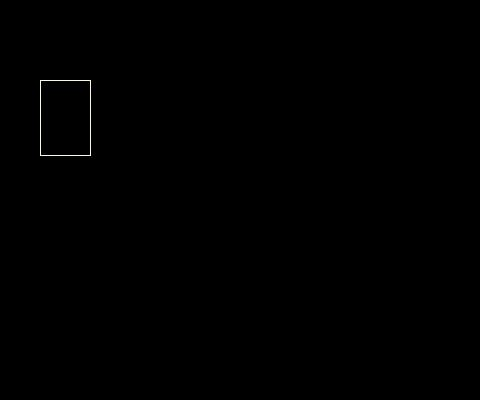• Another optional parameter is `fill`, if specified its value is to be the background color of the rectangle. For example,

x = 100

y = 20

w = 100

h = 25

xy = [ (x, y), (x + w, y + h) ]

canvas.rectangle( xy, fill='Lavender', outline='Orchid' )

Adds a filled-in rectangle to our drawing.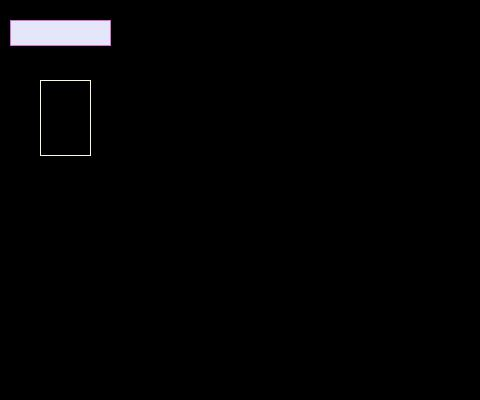• The function to draw an ellipse (oval) is `ImageDraw.ellipse()`. When drawing ellipses, the code specifies the upper-left-hand and lower-right-hand corners of the box that circumscribes the ellipse. * `fill` and `outline` parameters are optional parameters for `ImageDraw.oval()`
• The following code segment adds two ellipses to our drawing.

x = 120

y = 65

w = 150

h = 90

xy = [ (x, y), (x + w, y + h) ]

canvas.ellipse( xy, outline='Magenta' )

x = 300

y = 25

w = 50

h = 75

xy = [ (x, y), (x + w, y + h) ]

canvas.ellipse( xy, fill='DeepPink', outline='GhostWhite' )

Both ellipses have their perimeter drawn; and one also has its background coloorange red.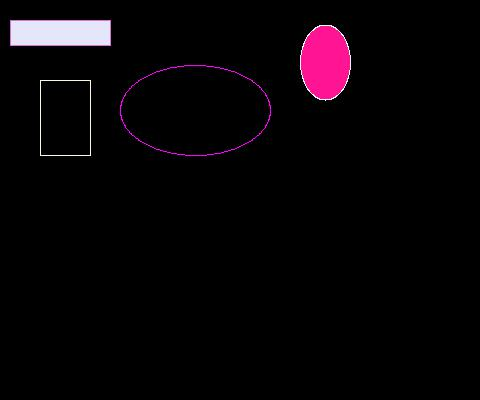• Pillow also provides the means to draw text through function `ImageDraw.text()`. The following code segment uses the function

coord = ( 25, 275 )

s = 'We are the best'

canvas.text( coord, s, fill='Moccasin' )

to add the string `'We are the best'` to our drawing. The first parameter to the function is the location to start the text; the second parameter is the desired text.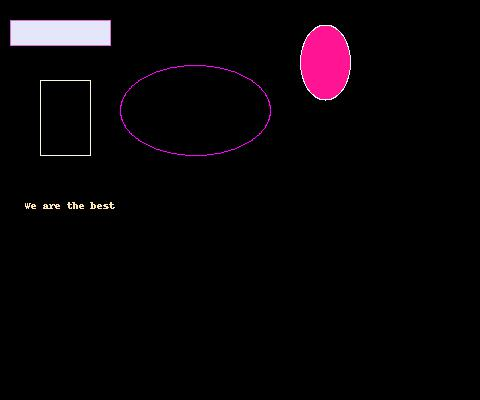• Another capability of `ImageDraw` is to draw a line through function `ImageDraw.line()`. The function requires an `xy` parameter and accepts optional parameters `fill` and `outline`. The following code segment

p1 = ( 25, 290 )

p2 = ( 115, 290 )

xy = [ p1, p2 ]

canvas.text( xy, s, fill='Moccasin' )

adds a horizontal line below our text.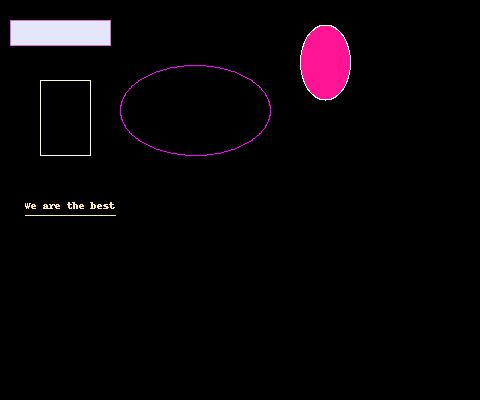• For drawing polygonal shapes `ImageDraw` has function `polygon()`. The function has one required parameter a list of locations `seq`. Optional parameters `fill` and `outline` are also available.
• The following code segment draws two polygons onto the canvas. The first polygon has its perimeter drawn; the second has its interior filled.

p1 = (350, 120)

p2 = (400, 140)

p3 = (425, 200)

p4 = (425, 260)

p5 = (375, 245)

p6 = (325, 190)

seq = [ p1, p2, p3, p4, p5, p6 ]

canvas.polygon( seq, outline='Peru' )

p1 = (175, 80)

p2 = (240, 110)

p3 = (190, 130)

p4 = (150, 120)

seq = [ p1, p2, p3, p4 ]

canvas.polygon( seq, fill='RebeccaPurple' )

• Afterwards the canvas now looks like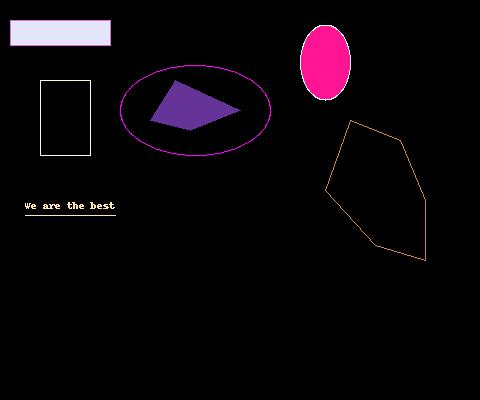• For drawing arcs of an ellipse use `ImageDraw` function `arc()`. The function has three required parameters `xy`, `a1`, and `a2`. Optional parameter `fill` can be used to specify the color of the arc. The function draws an arc within bounding box `xy` on the `canvas`. The arc outline has starting angle `a1` and ending angle `a2`.
• The following code segment adds a colored arc to the canvas.

p1 = ( 140, 220 )

p2 = ( 340, 380 )

xy = ( p1, p2 )

a1 = 0

a2 = 90

canvas.arc( xy, a1, a2, fill='RoyalBlue' )

im.save( 'arc.jpg' )

Note, a 0° angle points east; a 90° angle points south; a 180° angle points west; a 270° angle points north.

• Afterwards the canvas now looks like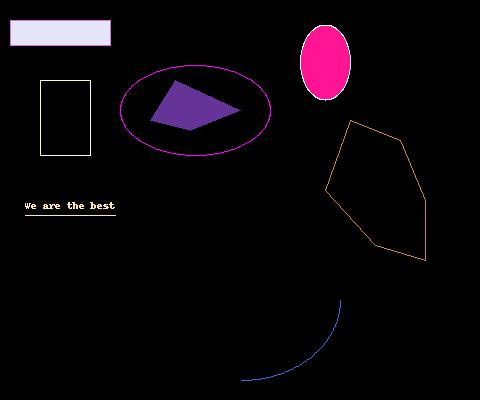• The `ImageDraw` function `chord()` supports the drawing of a chord for an ellipse. A cord connects to points on an ellipse with a straight line. Like `arc()`, the function has three required parameters `xy`, `a1`, and `a2`. Optional parameter `fill` can be used to specify the background of the chord; and optional parameter `outline` to color its perimeter.
• The following code segment adds a chord to the canvas.

p1 = ( 140, 220 )

p2 = ( 340, 380 )

xy = [ p1, p2 ]

a1 = 150

a2 = 210

canvas.chord( xy, a1, a2, fill='MediumTurquoise' )

• Afterwards, the canvas looks like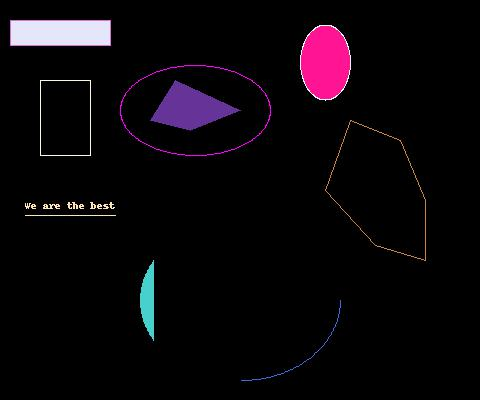• The `ImageDraw` function `pieslice()` supports the drawing of a pie slice for an ellipse. Like `arc()` and `chord()`, the function has three required parameters `xy`, `a1`, and `a2`. Optional parameter `fill` can be used to specify the background of the pie slice; and optional parameter `outline` to color its perimeter.
• The following code segment adds a pie slice to the canvas.

p1 = ( 140, 220 )

p2 = ( 340, 380 )

xy = [ p1, p2 ]

a1 = 225

a2 = 315

canvas.pieslice( xy, a1, a2, fill='OrangeRed' )

• Afterwards, the canvas looks like